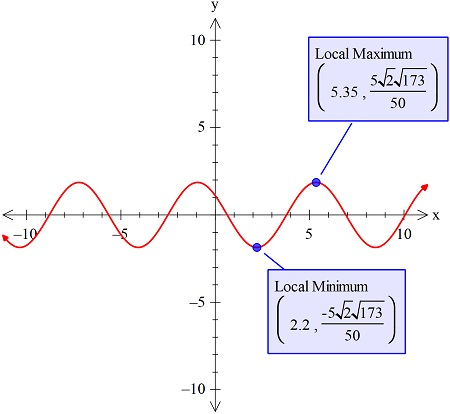# Let f ( x ) = 1.5 \sin ( x ) + 1.1 \cos ( x ) . What is the maximum and minimum value of...

## Question:

Let {eq}f ( x ) = - 1.5 \sin ( x ) + 1.1 \cos ( x ) {/eq}. What is the maximum and minimum value of this function?

## Minima & Maxima:

The curve will have the maxima or the minima or both, and that can be found with its graph. The highest point is the maxima but with the restricted domain there are many maxima or the minimas.

Here will plot the curve using the graphing utility and then using the graphing capability we will show the maxima and the minima points as shown in the figure below:So we have the maximum value of the function as:

{eq}\frac{\sqrt{356}}{10} {/eq}

and the minimum value is:

{eq}-\frac{\sqrt{356}}{10} {/eq}